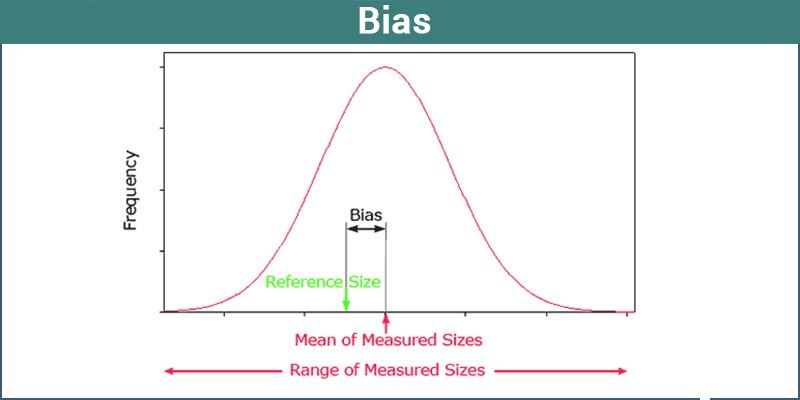# Statistical Term- Bias

Bias is a statistical term which means a systematic deviation from the actual value. It is a sampling procedure that may show some serious problems for the researcher as a mere increase cannot reduce it in sample size. Bias is the difference between the expected value and the real value of the parameter. In this article, we are going to discuss the classification of bias and its different types.## Bias Definition in Statistics

In statistics, bias is a term which defines the tendency of the measurement process. It means that it evaluates the over or underestimation of the value of the population parameter. Let us consider an example, in case you have the rule to evaluate the mean of the population. Hopefully, you might have found an estimation using the rule, which is the true reflection of the population. Now, by using the biased estimator, it is easy to find the difference between the true value and the statistically expected value of the population parameter.

## Types of Bias

The following are the different types of biases, which are listed below-

• Selection Bias
• Spectrum Bias
• Cognitive Bias
• Data-Snooping Bias
• Omitted-Variable Bias
• Exclusion Bias
• Analytical Bias
• Reporting Bias
• Funding Bias

## Classification of Bias

The bias is mainly categorized into two different types

### Measurement Bias

Measurement bias takes place for the duration of the carrying out the survey, and its consequences chiefly because of three reasons –

(i) The error happens while recording the data

While recording data, errors happen due to the malfunction of instruments that are used for data collection or because of ineffective handling of these tools by the researchers concerned with data collection.

The questions prepared for the survey might be put in a manner to lead the responses that are preferred by the researcher. There can be more choices for the preferred retort given than for the conflicting views.

(iii) Respondents gave inadvertent false responses

There can be a situation when many responders may have misunderstood the question and chooses an incorrect option. If the sample groups were composed of numerous senior citizens and if they asked to give answers by remembering their previous experiences, they might be providing some false inputs because of deficiency of memory.

### Non-Representative Sampling Bias

Non-representative sampling bias also referred to as selection bias. This inaccuracy occurs because of implementing random methods during the selection process. It results in an excess representation of some of the elements in the population. All the samples collected using convenience sampling are caused by bias. These type of situation are called under coverage bias.